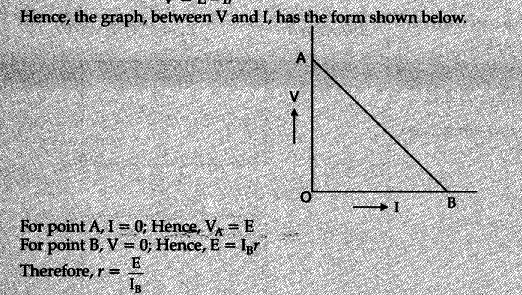# A cell of emf 'E' and internal reistance 'r' is connected across a variable resistor 'R'

A cell of emf ‘E’ and internal reistance ‘r’ is connected across a variable resistor ‘R’. Plot a graph showing variation of terminal voltage ‘V’ of the cell versus the current T. Using the plot, show that the emf of the cell and its internal resistance can be determined.

The relation between V and I is V = E-Ir